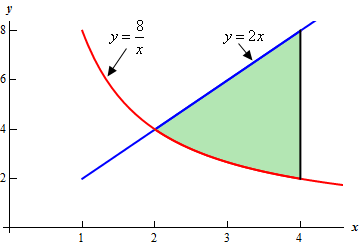Paul's Online Notes
Home / Calculus I / Applications of Integrals / Area Between Curves
Show Mobile Notice Show All Notes Hide All Notes
Mobile Notice
You appear to be on a device with a "narrow" screen width (i.e. you are probably on a mobile phone). Due to the nature of the mathematics on this site it is best views in landscape mode. If your device is not in landscape mode many of the equations will run off the side of your device (should be able to scroll to see them) and some of the menu items will be cut off due to the narrow screen width.

### Section 6.2 : Area Between Curves

4. Determine the area of the region bounded by $$\displaystyle y = \frac{8}{x}$$, $$y = 2x$$ and $$x = 4$$.

Show All Steps Hide All Steps

Hint : It’s generally best to sketch the bounded region that we want to find the area of before starting the actual problem. Having the sketch of the graph will usually help with determining the upper/lower functions and the limits for the integral.
Start Solution

Let’s start off with getting a sketch of the region we want to find the area of.

We are assuming that, at this point, you are capable of graphing most of the basic functions that we’re dealing with in these problems and so we won’t be showing any of the graphing work here.

Here is a sketch of the bounded region we want to find the area of.Show Step 2

For this problem we were only given one limit on $$x$$ (i.e. $$x = 4$$). To determine just what the region we are after recall that we are after a bounded region. This means that one of the given curves must be on each boundary of the region.

Therefore, we can’t use any portion of the region to the right of the line $$x = 4$$ because there will never be a boundary on the right of that region.

We also can’t take any portion of the region to the left of the intersection point. Because the first function is not continuous at $$x = 0$$ we can’t use any region that includes $$x = 0$$. Therefore, any portion of the region to the left of the intersection point would have to stop prior to the $$y$$-axis and any region like that would not have any of the given curves on the left boundary.

The region is then the one shown in graph above. We will take the region to the left of the line $$x = 4$$ and to the right of the intersection point.

Show Step 3

We now need to determine the intersection point. However, we should never just assume that our graph is accurate or that we will be able to read it accurately enough to guess the coordinates from the graph. This is especially true when the intersection point of the two curves does not occur on an axis (as they don’t in this case).

So, to determine the intersection point correctly we’ll need to find it directly. The intersection point is where the two curves intersect and so all we need to do is set the two equations equal and solve. Doing this gives,

$\frac{8}{x} = 2x\hspace{0.25in} \to \hspace{0.25in}{x^2} = 4\hspace{0.25in} \to \hspace{0.25in}x = - 2,\,\,\,\,x = 2$

Note that while we got two answers here the negative value does not make any sense because to get to that value we would have to go through $$x = 0$$ and as we discussed above the bounded region cannot contain $$x = 0$$.

Therefore the limits on $$x$$ are : $2 \le x \le 4$.

It should also be clear from the graph and the limits above that the upper function is $$y = 2x$$ and the lower function is $$y = \frac{8}{x}$$.

Step 3

At this point there isn’t much to do other than step up the integral and evaluate it.

We are assuming that you are comfortable with basic integration techniques so we’ll not be including any discussion of the actual integration process here and we will be skipping some of the intermediate steps.

The area is,

$A = \int_{2}^{4}{{2x - \frac{8}{x}\,dx}} = \left. {\left( {{x^2} - 8\ln \left| x \right|} \right)} \right|_2^4 = \require{bbox} \bbox[2pt,border:1px solid black]{{12 - 8\ln \left( 4 \right) + 8\ln \left( 2 \right) = 6.4548}}$